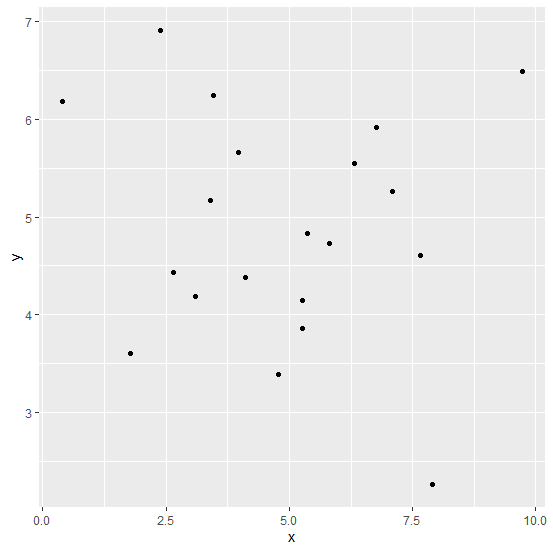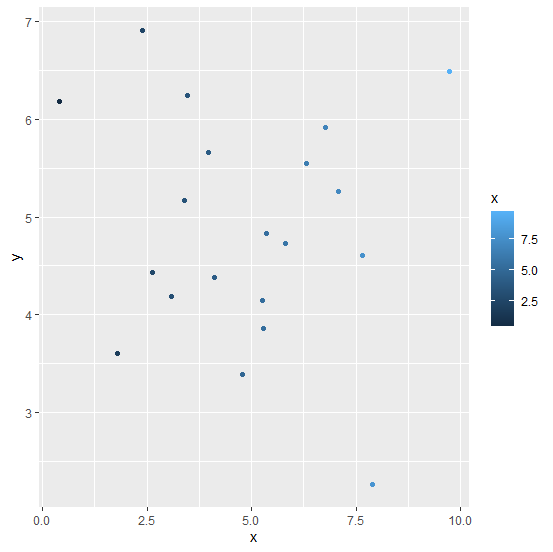# How to change the color of points in a scatterplot using ggplot2 in R?

To color the points in a scatterplot using ggplot2, we can use colour argument inside geom_point with aes. The color can be passed in multiple ways, one such way is to name the particular color and the other way is to giving a range or using a variable. If range or a variable will be used then the color of the points will be in different shades.

## Example

Live Demo

Consider the below data frame −

x<−rnorm(20,5,2)
y<−rnorm(20,5,1.25)
df<−data.frame(x,y)
df

## Output

      x       y
1 6.3184535 5.548867
2 3.4643698 6.247067
3 7.8930528 2.259042
4 7.6517535 4.606704
5 1.7838941 3.605288
6 0.3985215 6.183794
7 5.2738435 3.857376
8 4.7734419 3.389663
9 3.9727197 5.662962
10 3.3976335 5.172815
11 4.1068840 4.379264
12 6.7723590 5.914132
13 3.0944360 4.184177
14 7.0857100 5.266121
15 2.6391362 4.433864
16 5.2571231 4.144391
17 5.8119542 4.725406
18 5.3608015 4.828909
19 9.7308286 6.489042
20 2.3823201 6.916862

library(ggplot2)
ggplot(df,aes(x,y,col=x))+geom_point()

## OutputCreating the scatterplot by using the variable x −

## Example

ggplot(df,aes(x,y))+geom_point(aes(colour=x))

## Output